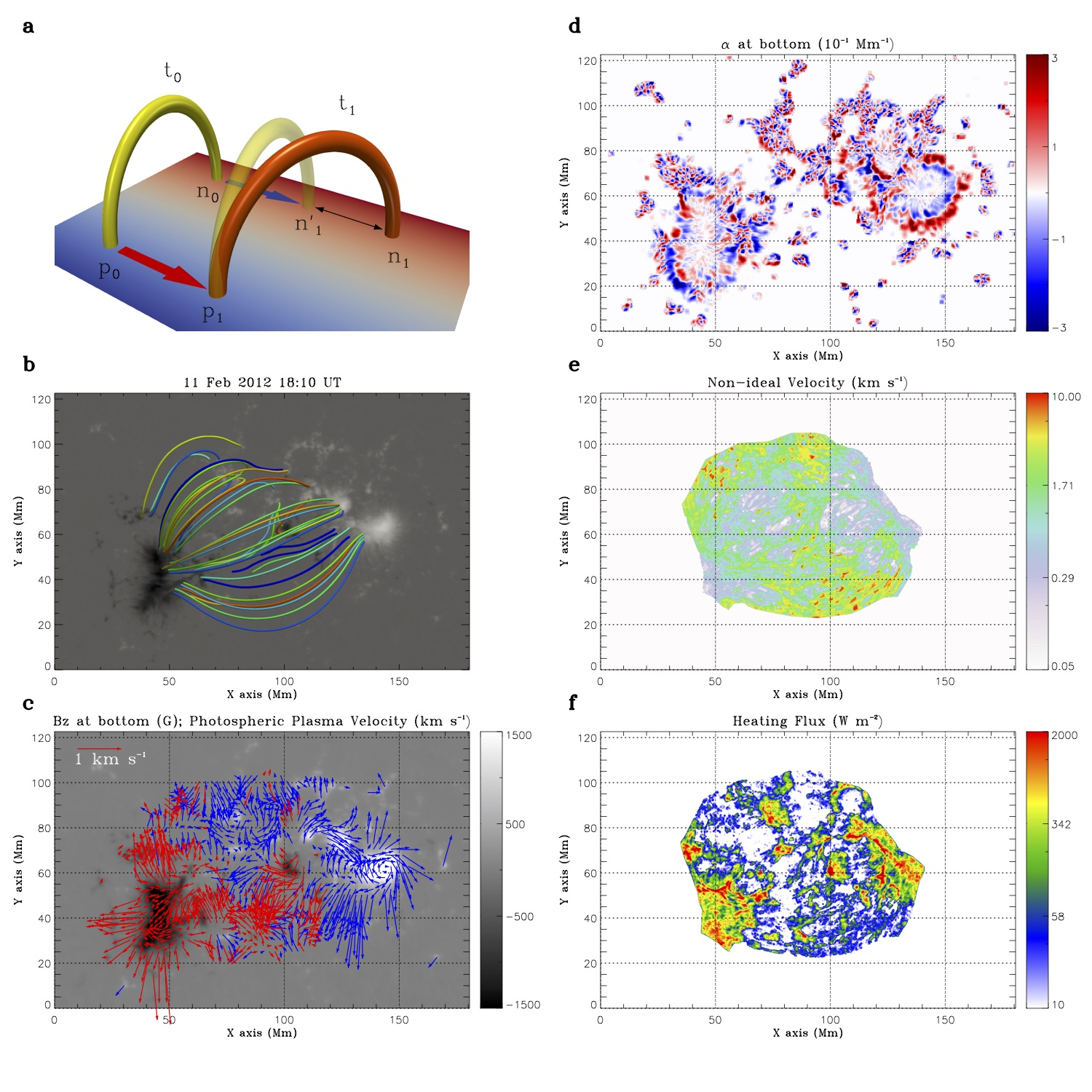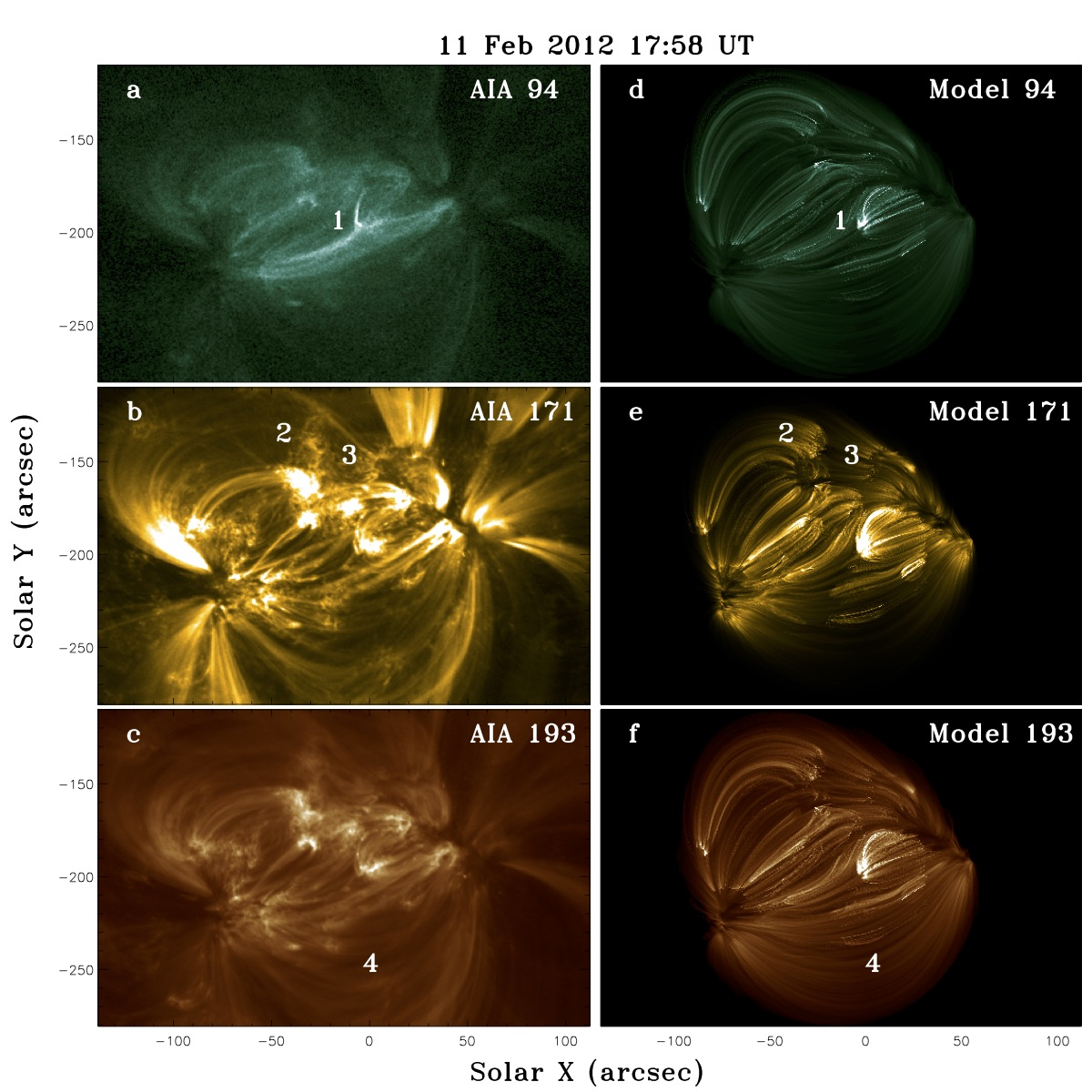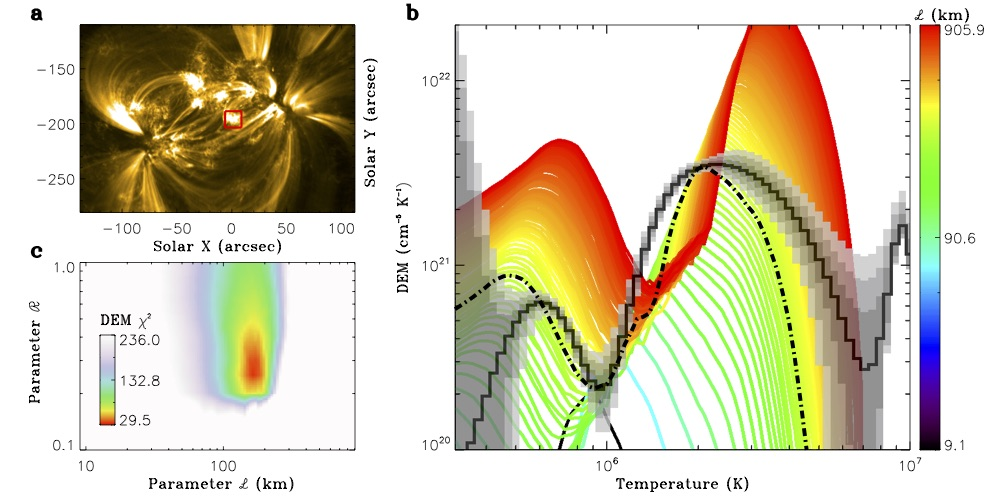# 100. Observationally Quantified Reconnection Providing a Viable Mechanism for Active Region Coronal Heating

Contributed by Kai E. Yang. Posted on June 5, 2018

Kai E. Yang1,2,3, Dana W. Longcope2, Yang Guo1,3, and M. D. Ding1,3
1. School of Astronomy and Space Science, Nanjing University, Nanjing, 210023, China
2. Physics Department, Montana State University, Bozeman, MT, 59717, USA
3. Key Laboratory for Modern Astronomy and Astrophysics (Nanjing University), Ministry of Education, Nanjing, 210023, China

Coronal heating remains one of the key problems to be solved in astrophysics. Magnetic reconnection is one of the proposed mechanisms that dissipate the required energy to heat the corona. In this work, we test this mechanism by measuring the heat flux delivered by magnetic reconnection with observed magnetograms.

In the absence of coronal reconnection, field-line footpoints move at the same velocity as the plasma they are rooted in. The rate of coronal reconnection is therefore related to any discrepancy between footpoint motion and local plasma motion, called non-ideal motion. We propose a novel method to measure this velocity discrepancy by combining a sequence of non-linear force-free field extrapolations with maps of photospheric velocity. We get both from a sequence of HMI vector magnetograms of active region NOAA 11416 on 11 February 2012. The measurement of the non-ideal velocity can be found in Figure 1.Figure 1| A model showing the non-ideal velocity and the measurement results. a, Coronal loops show the measurement of the non-ideal velocity. The letters p and n stand for the conjugate footpoints of a loop and the subscripts 0 and 1 refer to time labels for the start and end time, t0 and t1, respectively. The yellow loop represents the initial loop at t0 and the transparent yellow loop is the hypothetical version at time t1 under the assumption of ideal MHD. The footpoints are advected to new places by the photopsheric plasma flow, as indicated by the red and blue arrows in positive and negative polarities, respectively. The orange loop is the actual coronal loop at time t1. The distance denoted by the two-headed arrow is the non-ideal distance δn due to magnetic diffusion (reconnection). b, The extrapolated magnetic field at 18:10 UT. c, The photospheric plasma velocity field overplotted on the vertical magnetogram from HMI (background). The blue and red arrows represent the plasma flow of positive and negative magnetic flux, respectively. d, The force-free parameter, α, calculated from the vector magnetogram at 18:10 UT. e, The measured non-ideal velocity. Here, we only calculate the non- ideal velocity where both footpoints of the field lines are rooted within the field of view and |B| > 20 G in the magnetograms. f, The heating flux calculated from the reconnection model with the parameter ℒ = 160 km.

We build up the relationship between the non-ideal velocity and the heating due entirely to coronal re- connection. The heating rate can be expressed as$F=\frac{1}{\mu_{0}}\alpha\mathcal{L}v_s\bar{B}^2$, where α is the force-free parameter,vs is the non-ideal velocity, and ℒ is the diameter of the reconnected flux element. We fix it at 160 km in our model, which is consistent with the characteristic scale of the observed strands[2,3]. The result is shown in Figure 1f. This heating rate is used to predict density and temperature at points along an equilibrium loop. This in turn is used to synthesize emission in EUV (see Figure 2). Many corresponding structures can be found between them, e.g., the brightening loops, moss structures and large loops (indicated by numbers 1–4 in Figure 2).Figure 2| Observations and synthesized EUV images showing the loop structures. The left column (a, b, and c) shows the AIA observations at three channels. The right column (d, e, and f) shows the synthetic images at the same channels generated with ℒ = 160 km and ℛ = 0.3. All images use linear color scales with units of DN s−1, and corresponding pairs use the same color scale. The numbers indicate the corresponding structures, including brightening loops (1), moss structures (2 and 3), and large loops (4), respectively.

For the sensitivity analysis of the parameter ℒ and searching for the best fitting of the parameter ℛ, the ratio between the heating scale length and the loop length, we synthesize a column DEM over a subarea by varying the value of these two parameters (Figure 3). This is then compared with the DEM inverted directly from multi-channel AIA observations in the same subarea. This yields a discrepancy quantified by χ2 (Figure 3c). The results show that the choice of our parameters seems very reasonable.Figure 3| DEM distribution from the model and the discrepancy χ2 between the model and observations. a, The image at EUV 171 Å observed by AIA. The red box indicates the area where the DEM is calculated and shown in b. b, The DEM distribution calculated from the model by fixing ℛ = 0.3 but varying the scale length of the cross section of the loop, ℒ (see the color bar). The solid black curve is for the DEM inferred from the AIA observations at six wavelengths averaged over 12 minutes. The gray areas with different transparencies represent the results with 1σ, 2σ and 3σ from the Monte-Carlo test. The dash-dotted line is the curve from the model with ℒ = 160 km. c, The discrepancy χ2 between the modelled DEM and the observed one plotted as a color scale over parameter space.

In conclusion, we have developed a reconnection-based model to estimate the heating rate from the observed non-ideal velocity, which is derived from the HMI vector magnetograms. The model can predict the temperature and density distributions of the corona, at least to the first approximation. Our model avoids using an artificial high resistivity or specifying any form of dissipation at all. The predicted thermal structure of the corona, in particular the DEM and intensity distributions, resemble the observations both qualitatively and quantitatively. Thus, our study indicates that magnetic reconnection is a plausible heating mechanism to maintain an active region corona remarkably similar to the observed one.

For more details of this work, please refer to our recent publication: Kai E. Yang, et al., 2018, Nature Communications, 9, 692

### References

 Parker, E. N., 1998, ApJ, 330, 474
 Ji, H., Cao, W. & Goode, P. R., 2012, ApJL, 750, L25
 Cirtain, J. W. et al., 2013, Nature, 493, 501
 Rosner, R., Tucker, W. H. & Vaiana, 1978, ApJ, 220, 643

## 2 comments on “Observationally Quantified Reconnection Providing a Viable Mechanism for Active Region Coronal Heating”

1. Junwei Zhao

Wow! 100 science nuggets! Great achievement. Congratulations to the HMI science nugget team.